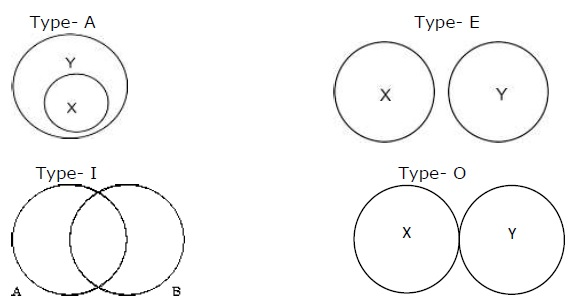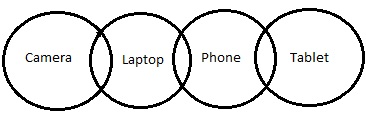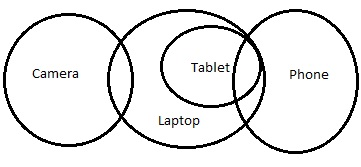# Reasoning - Syllogism

The meaning of syllogism as given by Greeks is Deduction. It’s a kind of logical argumentation.

### Definition of some important terms

There are some terms given below which have important role in solving problems on syllogism.

• Proposition − proposition is a sentence which makes statements. Proposition consists of 3 parts these are a) subject, b)predicate and c) the relation between subject and predicate. Some propositions are given below.

• All coasts are beaches.
• No students are honest.
• Some documents are secret.
• Subject and Predicate − Subject is that part about which something is being said. On the other hand, predicate is the part which is related to subject. For example − from the above propositions coasts, students, documents are subjects while beaches, honest, and secret are predicates.

### Types of Categorical Proposition

• Universal Proposition − Universal proposition is that which either includes subject fully or exclude it entirely. For example- No students are brainy. Universal proposition is further classified into following −

• Universal positive proposition − When the form is all X are Y then it is called positive proposition. It is generally denoted by letter A.

• Universal negative proposition − When the form is no X are T, then it is called as negative proposition. It is denoted by E.

• Particular proposition − It is the proposition that shows subject partially or exclude it partly but not completely. For instance, some cats are cotton. It is also divided into following types −

• Particular positive proposition − Forms like some X are U are called particular positive proposition. They are coded as I.

• Particular negative proposition − Forms like Some T are not P for Instance, some cats are not coils is called particular negative proposition and coded as O.

• Mediate inference − Here conclusion is taken from two statements. Example − “all lips are coils” and “all balls are bats”. So the conclusion will be “all lips are coils”.

• Immediate inference − Here conclusion will be taken from only one proposition. For instance, if the statement is “all teachers are god” then conclusion will be “some teachers are god”.

### Two important methods of immediate inference

These methods include conversion, contraposition etc.

• Implications − Suppose given that “all cats are beaches”, then it shows that the conclusion “some cats are beaches” is true. So if a given proposition is of A-category, then it also shows that I-type conclusion must be true.

• Conversion − It consists of 2 steps.

Step 1 − The subject will be converted into predicate and vice versa.

Step 2 − The proposition which is given will be changed accordingly.

For Example −

Statements − All the cats are racks. All the bags are racks. Some purses are bags.

Conclusions

1. Some cats are bags.

2. Some racks are cats.

3. Some racks are purses.

A - Only 1

B - Only 2 and 3

C - Only 1 and 2

D - Only 1 and 3

Solution −

Pictorial way of representing propositions are formulated by Euler. There are four ways in which the relation could be made according to four propositions.Type − A stands for “All X are Y”, Type − E stands for “No X are Y”, Type − I stands for “Some A are B” and Type − O stands for “Some X are not Y”.

For Example −

Statements −

Some cameras are laptops.

Some laptops are phones.

Some phones are tablets.

Conclusions −

I - At least some tablets are cameras

II - There is a possibility that all tablets are laptops

III - None of the tablets are laptop

Solution −

Circle Diagram −As per the above diagram,

Conclusion III follows. But ‘possibility’ word has been mentioned in the statement. So let’s check for the possibilities.

New diagram will be −But in this case, conclusion III does not follow. Either conclusion II or conclusion III follows.

reasoning_syllogism.htm